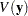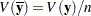## Example 57.2 Reading Means and Covariance Matrices from a DATA= COV Data Set

This example creates a COV-type data set that contains sample means and covariance matrices computed from imputed data sets. These estimates are then combined to generate valid statistical inferences about the population means.

The following statements use the CORR procedure to generate sample means and a covariance matrix for the variables in each imputed data set:

```proc corr data=outmi cov nocorr noprint out=outcov(type=cov);
var Oxygen RunTime RunPulse;
by _Imputation_;
run;
```

The following statements display (in Output 57.2.1) output sample means and covariance matrices from PROC CORR for the first two imputed data sets:

```proc print data=outcov(obs=12);
title 'CORR Means and Covariance Matrices'
' (First Two Imputations)';
run;
```

Output 57.2.1 COV Data Set
 CORR Means and Covariance Matrices (First Two Imputations)

Obs _Imputation_ _TYPE_ _NAME_ Oxygen RunTime RunPulse
1 1 COV Oxygen 28.5603 -7.2652 -11.812
2 1 COV RunTime -7.2652 2.5214 2.536
3 1 COV RunPulse -11.8121 2.5357 79.271
4 1 MEAN   47.0120 10.4441 171.216
5 1 STD   5.3442 1.5879 8.903
6 1 N   31.0000 31.0000 31.000
7 2 COV Oxygen 27.1240 -6.6761 -10.217
8 2 COV RunTime -6.6761 2.2035 2.611
9 2 COV RunPulse -10.2170 2.6114 95.631
10 2 MEAN   47.2407 10.5040 171.244
11 2 STD   5.2081 1.4844 9.779
12 2 N   31.0000 31.0000 31.000

Note that the covariance matrices in the data set outcov are estimated covariance matrices of variables,. The estimated covariance matrix of the sample means is, whereis the sample size, and is not the same as an estimated covariance matrix for variables.

The following statements combine the results for the imputed data sets, and derive both univariate and multivariate inferences about the means. The EDF= option is specified to request that the adjusted degrees of freedom be used in the analysis. For sample means based on 31 observations, the complete-data error degrees of freedom is 30.

```proc mianalyze data=outcov edf=30;
modeleffects Oxygen RunTime RunPulse;
run;
```

The "Variance Information" and "Parameter Estimates" tables display the same results as in Output 57.1.2 and Output 57.1.3, respectively, in Example 57.1.

With the WCOV, BCOV, and TCOV options, as in the following statements, the procedure displays the between-imputation covariance matrix, within-imputation covariance matrix, and total covariance matrix assuming that the between-imputation covariance matrix is proportional to the within-imputation covariance matrix in Output 57.2.2.

```proc mianalyze data=outcov edf=30 wcov bcov tcov mult;
modeleffects Oxygen RunTime RunPulse;
run;
```

Output 57.2.2 Covariance Matrices
The MIANALYZE Procedure

Within-Imputation Covariance Matrix
Oxygen RunTime RunPulse
Oxygen 0.930852655 -0.226506411 -0.461022083
RunTime -0.226506411 0.073141598 0.080316017
RunPulse -0.461022083 0.080316017 3.114441784

Between-Imputation Covariance Matrix
Oxygen RunTime RunPulse
Oxygen 0.0414778123 0.0099248946 0.0183701754
RunTime 0.0099248946 0.0029478891 0.0091684769
RunPulse 0.0183701754 0.0091684769 0.1910855259

Total Covariance Matrix
Oxygen RunTime RunPulse
Oxygen 1.202882661 -0.292700068 -0.595750001
RunTime -0.292700068 0.094516313 0.103787365
RunPulse -0.595750001 0.103787365 4.024598310

With the MULT option, the procedure assumes that the between-imputation covariance matrix is proportional to the within-imputation covariance matrix and displays a multivariate inference for all the parameters taken jointly.

Output 57.2.3 Multivariate Inference
Multivariate Inference
Assuming Proportionality of Between/Within Covariance Matrices
Avg Relative
Increase
in Variance
Num DF Den DF F for H0:
Parameter=Theta0
Pr > F
0.292237 3 122.68 12519.7 <.0001

The "Multivariate Inference" table in Output 57.2.3 shows a significant-value for the null hypothesis that the population means are all equal to zero.